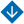Constructing Power-Exponential and Linear-Logarithmic Regression Models
•About Advisory & Editorial Boards Aims & Scope Publication Ethics Review Process Archive Contact Information

#Constructing Power-Exponential and Linear-Logarithmic Regression Models

Bazilevskiy, M.P. Constructing Power-Exponential and Linear-Logarithmic Regression Models

Abstract. When using nonlinear regression models, the estimates of the resulting dependence are often difficult or even impossible to interpret. This paper develops nonlinear regression specifications in which any estimated parameter, except the free term, can always be given some practical interpretation. A multiplicative power-exponential regression generalizing the Cobb–Douglas production function and an additive linear-logarithmic regression are constructed. Three construction strategies are formulated for each of them, and the issues of interpreting their estimates are considered in detail. The construction strategies based on the least absolute deviations method are formalized as linear and partially Boolean linear programming problems. The mathematical apparatus developed in this paper is illustrated by modeling rail freight traffic in Irkutsk oblast.

Keywords: regression model, interpretation, multiplicative power-exponential regression, linear-logarithmic regression, feature selection, least absolute deviations, rail freight traffic.

PDF (English)